# 求 pi 的值

1777年，法国数学家布丰（Georges Louis Leclere de Buffon，1707—1788）提出用投针实验的方法求圆周率π。

## 基本原理概述

PI/4:1 = c:n
PI = 4*c/n


## java 代码实现

public void calcPiTest() {
final int N = 100000;
int count = 0;
for (int i = 1; i < N; i++) {
double x = Math.random();
double y = Math.random();
if ((x * x + y * y) < 1) {
count++;
}
}
double pi = (double) 4 * count / N;
System.out.println("PI = " + pi);
}


PI = 3.14588


ps: 我尝试了提升测试的次数，其实结果并没有变得更加精确，猜测是随机数的问题。

# 蒙特卡罗积分

## 解决方式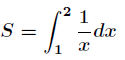## 蒙特卡洛积分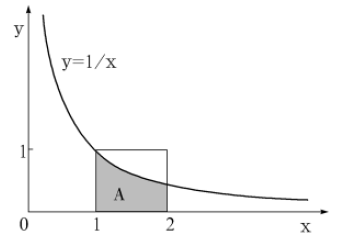(x1,y1), (x2,y2), ..., (xn,yn)


1/xi ≥ yi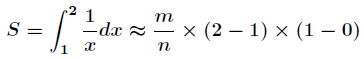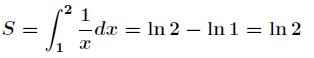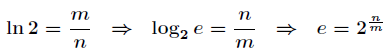## java 编码

int max = 100000;
int count = 0;
for (int i = 1; i < max; i++) {
// 落在阴影之中
if ((x * y) <= 1.0) {
count++;
}
}

double e = Math.pow(2.0, 1.0*max/count);
System.out.println(e);


• 输出
2.7199979218512764


# 拓展阅读

《算法之美：隐匿在数据结构背后的原理》